# SOCR EduMaterials AnalysisActivities OneZ

## SOCR Analysis - SOCR Analyses Example on One Sample Z-Test

This SOCR Activity demonstrates the utilization of the SOCR Analyses package for statistical Computing. In particular, it shows how to use SOCR One Sample Z-Test.

## One Sample Z-Test Example

Go to the SOCR One-Sample Z-Test Applet. We will demonstrate One Sample Z-Test with some SOCR built-in examples, however, you can copy-paste in, or load your own data in the applet.

This example is based on the data taken from the statistical program "R." For more information of the R program, please see CRAN Home Page. The dataset used here is "shoes" under R's "MASS" library. In the dataset, ten boys are given to the wear of shoes of materials A and B for one foot. The worn level is recorded and difference is obtained. We'd like to find if one material is better than the other. The difference is calculated by B - A. (Note: this example is also used in another three SOCR demonstration: Two Paired Sample T-Test, Two Paired Sample Sign Test, and Two Paired Sample Signed Rank Test. If you'd like to see the data of each material, go to the page of these activities or directly look for them in the SOCR Applet.

### Select the One Sample Z-test

Click on One Sample Z-Test at the left panel's combo box.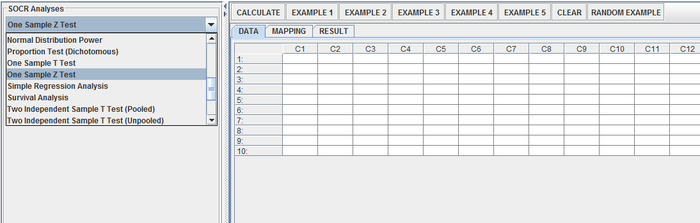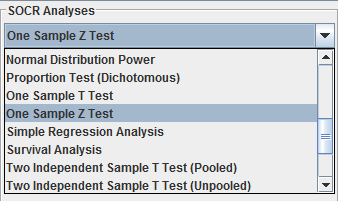On the right panel, first click on EXAMPLE 1 and next click on "Data" to retrieve the demonstrated example. This is the example we'll be looking at. Note that the data are difference of B - A.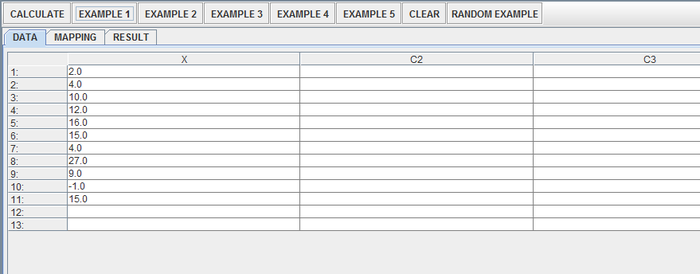### Mapping

Click on the "Mapping" button to get to the "Mapping" panel. Click on "ADD" under VARIABLE to add the variable for One Sample Z-Test. Here, the Column X = B - A. Don't worry about C2 to C3.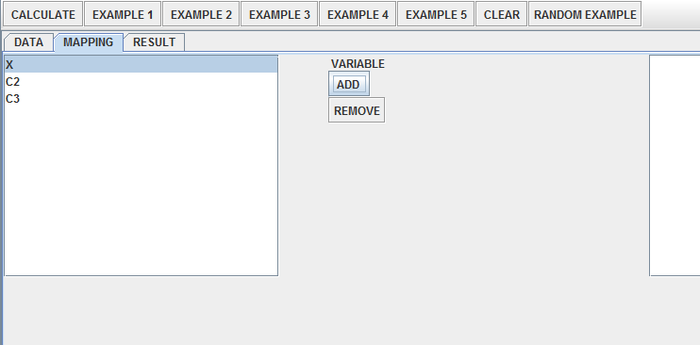### Calculate

Click on "Calculate" – a dialogue box will pop up and ask for the test (population) mean. Here we use a test mean of 12; if no input is entered, the default would be zero. After inputting the test mean, another box will pop up and ask for test variance. Here we use a test variance of 50; if no input is entered, the default would be one. The user will then be able to see the result under “Result” tab.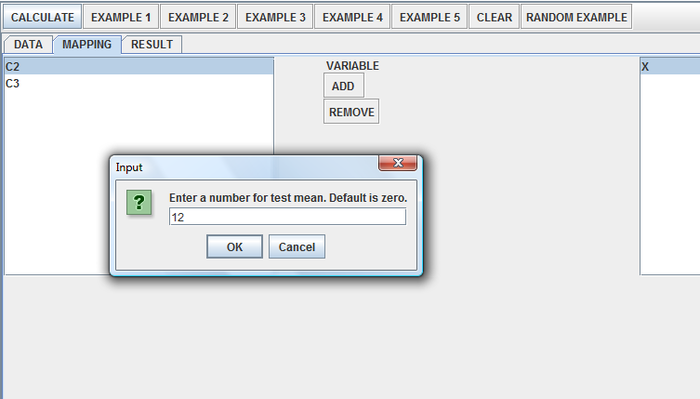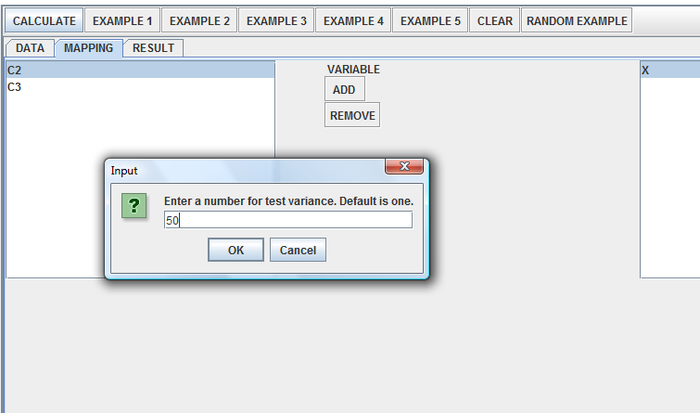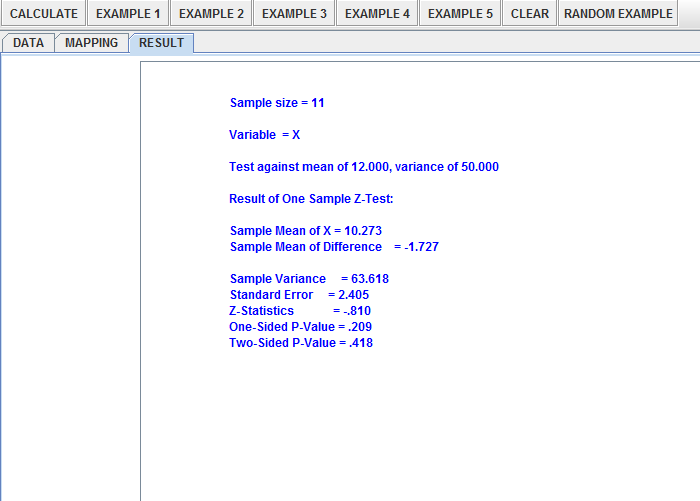### Questions

• By looking at the test results, what do you think about the data?
• What can you say about the two different shoe materials?